American Journal of Electrical Power and Energy Systems
Volume 4, Issue 6-1, December 2015, Pages: 8-12

Comparative Analysis of Hysteresis and Fuzzy-Logic Hysteresis Current Control of a Single-Phase Grid-Connected Inverter

Mihail Antchev1, Angelina Tomova-Mitovska2

1Power Electronics Department, Faculty of Electronic Engineering and Technologies, Technical University- Sofia, Sofia, Bulgaria

2Schneider Electric Slovakia, Bratislava, Slovakia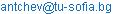(M. Antchev)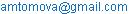(A. Tomova-Mitovska)

Mihail Antchev, Angelina Tomova-Mitovska. Comparative Analysis of Hysteresis and Fuzzy-Logic Hysteresis Current Control of a Single-Phase Grid-Connected Inverter. American Journal of Electrical Power and Energy Systems. Special Issue: Improvement of Energy Efficiency in the Conversion of Electrical Energy by Means of Electronic Converters. Vol. 4, No. 6-1, 2015, pp. 8-12. doi: 10.11648/j.epes.s.2015040601.12

Abstract: This paper presents a comparative analysis of two current control methods of single phase on-grid inverter- the hysteresis current control and the fuzzy logic hysteresis current control method. The aim of the paper is to compare the values of the main researched parameters of the on-grid inverter and to show which control method is more energy efficient. The research model is established by means of differential equations with the software MATLAB/SIMULINK. The comparison of the operation of the inverter with both control methods is analyzed in two different cases of the output power of the system - 800W and 1600W approximately.

Keywords: Hysteresis Current Control, Fuzzy Logic, On-Grid Inverter

Contents

1. Introduction

The most frequently used methods of control of on-grid inverter are the Pulse-Width Modulation (PWM) method and the hysteresis current control method. Both of them have advantages as well as disadvantages , . One more recent and innovative method combines the mathematic concept of fuzzy logic by means of which uncertain and vague problems can be solved  with hysteresis current control , .

Fuzzy logic is a mathematic concept which allows the solution of not very precise problems and it is based on incertitude and vagueness . This logic is used in the control of on-grid inverters .

The other control logic used in this paper- the hysteresis current control is based on creation of reference signal which is compared constantly with the inverter output signal. This method is applied in different power electronic devices- on-grid inverters , , active power filters , bi-directional converters .

An example of the combination of both methods- fuzzy logic and hysteresis current control for active power filters is presented in , .

This paper presents a comparative analysis of hysteresis and fuzzy logic hysteresis current control of on-grid inverter. Both models are developed in the MATLAB/SIMULINK environment and use the same on-grid inverter model realized on the basis of solving of differential equations connected to the grid via a LCL-filter. In the case of classical hysteresis current control the hysteresis value is constant and in the case of the combined fuzzy logic and hysteresis current control method, three different values of the hysteresis are used according to the current value of the difference between the transitory values of the current of the inverter and the reference current.

The operation of the inverter is studied in two cases. In the first case the power of the inverted is sufficient to supply the load and in the second case its power is lower than the desired power by the load.

2. Operation

In the first case the inverter control based on hysteresis current control uses as a feedback signal a comparison of two signals- the voltage of the point of common coupling VPCC and the inverter current IINV. Voltage is needed for the synchronization. The current is compared to a preliminary set reference current iREF. The result of the comparison of the difference of these two currents and the hysteresis H defines which couple of power switches will be turned on .

In the second case when the control of the inverter is based on combined fuzzy logic and hysteresis current control, the fuzzy logic is implemented by comparison of the transitory values of the inverter output current and reference current to produce output signal based on fuzzy logic by choosing one of the three values of the hysteresis .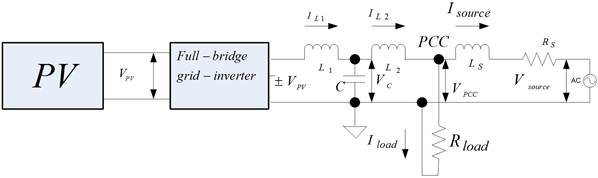Figure 1. Block diagram of the system.

2.1. Input Data

For the simulation of the model the following data is used: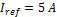;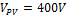;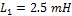;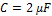;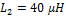;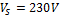;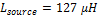;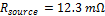;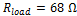, corresponding to an output power of 778W and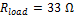, corresponding to an output power of 1603W.

The value of the hysteresis in the hysteresis-current control is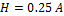.

The three values of the hysteresis of the fuzzy-logic current control are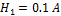,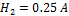,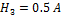.

For the needs of the software currents, voltages and hysteresis values are lessened by the factor of 100, in order to be operated in the range of the variables of MATLAB/SIMULINK.

2.2. Equations

The equations used to create the model of the system are: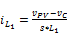(1)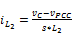(2)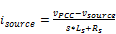(3)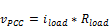(4)

whereis the Laplace’s operator.

The fuzzy-logic based equations are described here below:

if (iL1≥H1| iL1≥H2| iL1≥H3 ) { -1;}else if (iL1≤H1| iL1≤H2| iL1≤H3){1;}                            (5)

2.3. Simulation Models

2.3.1. Hysteresis-Current Control Model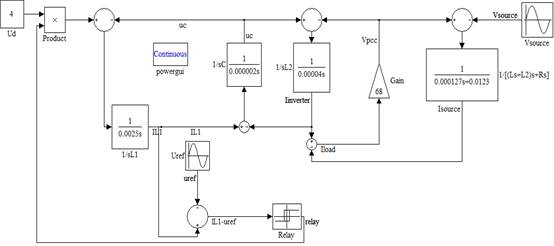Figure 2. Simulation of hysteresis control of single-phase grid inverter.

2.3.2. Fuzzy-Logic Hysteresis-Current Control ModelFigure 3. Simulation of fuzzy logic hysteresis control of single-phase grid inverter.

3. Results of the Computer Simulation

3.1.1. Waveforms

In this case the power of the inverter is sufficient to supply the load.

From the two sets of waveforms on Fig.4 one can notice identical waveforms. In both cases the load current is in phase with the voltage at the point of common coupling which is normal as the load is resistive. On the other hand the inverter current is displaced in 180° to the voltage at the common point of coupling.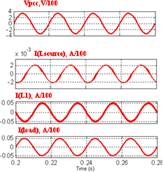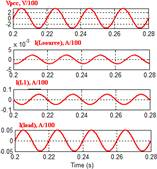(a) (b) Figure 4. Waveforms obtained from the simulation of the on-grid inverter model. Hysteresis current control on the left diagrams and fuzzy logic hysteresis current control on the right diagrams. From top to bottom: the voltage of the PCC, the grid current, the inverter current, the load current: a) hysteresis current control results; b) fuzzy-logic hysteresis-current control.

From the results we can notice that both methods of control have the same efficiency as the waveforms have the same shapes and values.

3.1.2. Frequency Analysis

Frequency analysis of the voltage at the point of common coupling (VPCC) and inverter current (IL) for both control methods are presented in Fig.5.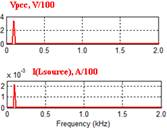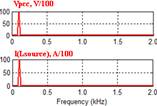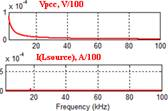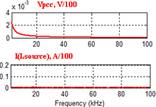(a) (b) Figure 5. Harmonic spectrum of the voltage of PCC- upper diagram and source current- lower diagram. Hysteresis current control on the left diagrams and fuzzy logic hysteresis current control on the right diagrams. The X-axis span from 0 to 2kHz (four upper co-ordinate systems) and from 2kHz to 100kHz (four lower co-ordinate systems).

The results are divided in two joint co-ordinate systems- from 0 to 2 kHz and from 2kHz to 100 kHz.

The shapes of the simulation results for both methods are identical. However we can notice higher values of the main harmonic for VPCC and IL for the combined fuzzy logic hysteresis control method.

3.2.1. Waveforms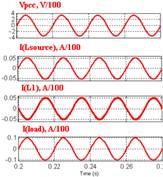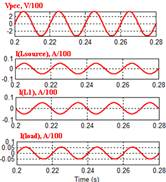(a) (b) Figure 6. Waveforms obtained from the simulation of the on-grid inverter model. Hysteresis current control on the left diagrams and fuzzy logic hysteresis current control on the right diagrams. From top to bottom: the voltage of the PCC, the grid current, the inverter current, the load current: a) hysteresis current control results; b) fuzzy-logic hysteresis-current control.

In this case the power of the inverter is not sufficient to supply the load and the grid supplies half of the active power.

From the two sets of waveforms presented in Fig.6 one can notice identical waveforms as in the case of load of 68Ω. In both cases the load current is in phase with the voltage at the point of common coupling which is normal as the load is resistive. On the other hand the inverter current is displaced in 180° to the voltage at the common point of coupling.

As in the previous case from Fig.4 both methods of control have the same efficiency as the waveforms have the same shapes and values.

3.2.2. Frequency Analysis

Frequency analysis is done the same way as in the previous case. Results are presents in Fig.7.

In this case when power of the inverter is not sufficient to supply the load and half of the active power is supplied by the grid we can notice the same phenomena as in the case when the power of the inverter was sufficient to supply the load- same shapes, same harmonics, higher values for the combined method of control.

The one remarkable difference is the lack of harmonics for the higher harmonics in the case of the combined control, which is not the case for the hysteresis control results.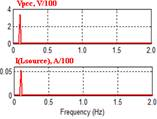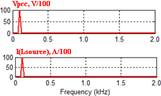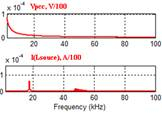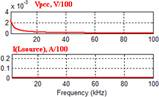(a) (b) Figure 7. Harmonic spectrum of the voltage of PCC- upper diagram and source current-lower diagram. Hysteresis current control on the left diagrams and fuzzy logic hysteresis current control on the right diagrams. The X-axis span from 0 to 2kHz (four upper co-ordinate systems) and from 2kHzto 100kHz (four lower co-ordinate systems).

4. Conclusion

The comparative analysis of two methods of control of on-grid inverters presented in this paper proved the advantages of each of them.

From the simulation results in the time and frequency domain it is clear that both methods could provide correct control of the on-grid inverter so that it can operates in parallel with the electric grid.

The results for the shapes and values of the main parameters of the system for both control methods are identical. The only difference can be noticed in the frequency analysis simulations- where in both cases harmonic values of the study for the combined method are higher.

This paper presents only computer simulation results but they are significant enough to show that in case of a study on system or grid which is more sensitive to harmonics or which have some special requirements for the high harmonics we should use the hysteresis current control to do the study.

In case of no special requirements regarding the high harmonics and physical realization of the system we can use the combined method as it is easier to be implemented.

References

1. H. Mao, X. Yang, Z. Chen, Z. Wang, "A hysteresis current controller for single-phase three-level voltage source inverters," IEEE Transactions on Power Electronics, vol. 27, no. 7, pp. 3330-3339, 2012.
2. M. Kazmierkowski, L. Malesani, "Current control techniques for three-phase voltage-source PWM converters," IEEE Transactions on Industrial Electronics, vol. 45, no. 5, pp. 691-703, 1998.
3. C. C. Lee, "Fuzzy logic control systems: Fuzzy logic controller, Part II," IEEE Transactions on Systems, Man and Cybernetics, vol. 20, no. 2, pp. 419-435, 1990.
4. Z. Yao,L. Xiao, "Two-switch dual-buck grid-connected inverter with hysteresis current control," IEEE Transactions on Power Electronics, vol. 27, no. 7, pp. 3310-3318, 2012
5. Y. Ounejjar, K. Al-Haddad, L. A. Dessaint, "A novel six-band hysteresis control for the packed U cells seven-level converter: Experimental validation," IEEE Transactions on Industrial Electronics, vol. 50, no. 10, pp. 3808-3816, 2012
6. C. C. Lee, "Fuzzy logic control systems: Fuzzy logic controller, Part II," IEEE Transactions on Systems, Man and Cybernetics, vol. 20, no. 2, pp. 419-435, 1990.
7. I. Sefa,N. Altin, "Simulation of fuzzy logic controlled grid interactive inverter," Univeristy of Pitesti- Electronics and Computer Science, Scientific Bulletin, vol. 2, no. 8, pp. 30-35, 2008.
8. Z. Yao,L. Xiao, "Two-switch dual-buck grid-connected inverter with hysteresis current control," IEEE Transactions on Power Electronics, vol. 27, no. 7, pp. 3310-3318, 2012.
9. J. Bauer,J. Lettl, "Solar power station output inverter control design," Radioengineering, vol. 20, no. 1, pp. 258-262, 2011.
10. M. H. Antchev, M. P. Petkova, A. Kostov, "Hysteresis current control of single-phase shunt active power filter using frequency limitation," in Ninth IASTED Conference Power and Energy Systems, 2007, pp. 234-238.
11. M. Makhlouf, F. Messai, H. Benalla, "Modeling and control of a single-phase grid connected photovoltaic system," Journal of Theoretical and Applied Information Technology, vol. 37, no. 2, pp. 289-296, 2012.
12. Petkova M., M. Antchev, A. Tomova, "Computer investigation and verification of the operation of single-phase on-grid inverter with hysteresis current control" in Proc. EUROCON 2013, 1-4 July 2013, Zagreb, Croatia, pp.976-983, (978-1-4673-2232-4/13)
13. Tomova A., M. Antchev, M. Petkova, H. Antchev, "Fuzzy Logic Hysteresis Control of А Single-Phase on-Grid Inverter: Computer Investigation", International Journal of Power Electronics and Drive System (IJPEDS), Vol. 3, No. 2, June 2013, pp. 1~8, ISSN: 2088-8694

 Contents 1. 2. 2.1. 2.2. 2.3. 3. 3.1. 3.2. 4.
Article ToolsAbstractPDF(360K)